# Forecast Accuracy[Page 683 ( continued )]

It is not probable that a forecast will be completely accurate; forecasts will always deviate from the actual demand. This difference between the forecast and the actual is referred to as the forecast error . Although some amount of forecast error is inevitable, the objective of forecasting is for the error to be as slight as possible. Of course, if the degree of error is not small, this may indicate either that the forecasting technique being used is the wrong one or that the technique needs to be adjusted by changing its parameters (for example, a in the exponential smoothing forecast).

The forecast error is the difference between the forecast and actual demand .

[Page 684]

There are a variety of different measures of forecast error, and in this section we discuss several of the most popular ones: mean absolute deviation ( MAD ), mean absolute percent deviation ( MAPD ), cumulative error ( E ), average error or bias (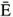), and mean squared error ( MSE ).

#### Mean Absolute Deviation

The mean absolute deviation (MAD) is one of the most popular and simplest-to-use measures of forecast error. MAD is an average of the difference between the forecast and actual demand, as computed by the following formula: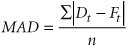where

 t = the period number D t = demand in period t F t = the forecast for period t n = the total number of periods = the absolute value

MAD is the average, absolute difference between the forecast and the demand .

In our examples for PM Computer Services, forecasts were developed using exponential smoothing (with a = .30 and with a = .50), adjusted exponential smoothing ( a = .50, b = .30), and a linear trend line for the demand data. The company wants to compare the accuracy of these different forecasts by using MAD .

We will compute MAD for all four forecasts; however, we will only present the computational detail for the exponential smoothing forecast with a = .30. Table 15.8 shows the values necessary to compute MAD for the exponential smoothing forecast.

##### Table 15.8. Computational values for MAD and error

Period

Demand, D t

Forecast, F t ( a = .30)

Error ( D t F t )

D t F t

Error 2 ( D t F t ) 2

1

37

37.00

2

40

37.00

3.00

3.00

9.00

3

41

37.90

3.10

3.10

9.61

4

37

38.83

1.83

1.83

3.35

5

45

38.28

6.72

6.72

45.15

6

50

40.29

9.71

9.71

94.28

7

43

43.20

0.20

0.20

0.04

8

47

43.14

3.86

3.86

14.90

9

56

44.30

11.70

11.70

136.89

10

52

47.81

4.19

4.19

17.56

11

55

49.06

5.94

5.94

35.28

12

54

50.84

3.16

3.16

9.98

520 [*]

49.31

53.41

376.04

[*] Because the computation of MAD will be based on the 11 periods 2 through 12, S D t = 520, excluding the demand value of 37.

Using the data in Table 15.8, MAD is computed as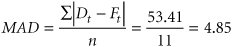[Page 685]

In general, the smaller the value of MAD , the more accurate the forecast, although, viewed alone, MAD is difficult to access. In this example, the data values were relatively small, and the MAD value of 4.85 should be judged accordingly . Overall, it would seem to be a "low" value (i.e., the forecast appears to be relatively accurate). However, if the magnitude of the data values were in the thousands or millions, then a MAD value of a similar magnitude might not be bad either. The point is that you cannot compare a MAD value of 4.85 with a MAD value of 485 and say the former is good and the latter is bad; they depend to a certain extent on the relative magnitude of the data.

The lower the value of MAD relative to the magnitude of the data, the more accurate the forecast .

One benefit of MAD is being able to compare the accuracy of several different forecasting techniques, as we are doing in this example. The MAD values for the remaining forecasts are

• Exponential smoothing ( a = .50): MAD = 4.04

• Adjusted exponential smoothing ( a = .50, b = .30): MAD = 3.81

• Linear trend line: MAD = 2.29

When we compare all four forecasts, the linear trend line has the lowest MAD value, of 2.29. It would seem to be the most accurate, although it does not appear to be significantly better than the adjusted exponential smoothing forecast. Furthermore, we can deduce from these MAD values that increasing a from .30 to .50 enhanced the accuracy of the exponentially smoothed forecast. The adjusted forecast is even more accurate.

A variation of MAD is the mean absolute percent deviation (MAPD) . It measures the absolute error as a percentage of demand rather than per period. As a result, it eliminates the problem of interpreting the measure of accuracy relative to the magnitude of the demand and forecast values, as MAD does. MAPD is computed according to the following formula: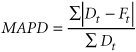MAPD is absolute error as a percentage of demand .

Using the data from Table 15.8 for the exponential smoothing forecast ( a = .30) for PM Computer Services, MAPD is computed as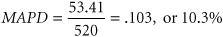A lower MAPD implies a more accurate forecast. The MAPD values for our other three forecasts are

• Exponential smoothing ( a = .50): MAPD = 8.5%

• Adjusted exponential smoothing ( a = .50, b = .30): MAPD = 8.1%

• Linear trend line: MAPD = 4.9%

#### Cumulative Error

Cumulative error is computed simply by summing the forecast errors, as shown in the following formula:

E =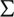e t

Cumulative error is the sum of the forecast errors .

A relatively large positive value indicates that the forecast is probably consistently lower than the actual demand or is biased low. A large negative value implies that the forecast is consistently higher than actual demand or is biased high. Also, when the errors for each period are scrutinized and there appears to be a preponderance of positive values, this shows that the forecast is consistently less than actual, and vice versa.

[Page 686]

The cumulative error for the exponential smoothing forecast ( a = .30) for PM Computer Services can be read directly from Table 15.8; it is simply the sum of the values in the "Error" column:

E =e t = 49.31

This relatively large-value positive error for cumulative error and the fact that the individual errors for each period in Table 15.8 are positive indicate that this forecast is frequently below the actual demand. A quick glance back at the plot of the exponential smoothing ( a = .30) forecast in Figure 15.3 visually verifies this result.

The cumulative errors for the other forecasts are

• Exponential smoothing ( a = .50): E = 33.21

• Adjusted exponential smoothing ( a = .50, b = .30): E = 21.14

We did not show the cumulative error for the linear trend line. E will always be near zero for the linear trend line; thus, it is not a good measure on which to base comparisons with other forecast methods .

A measure closely related to cumulative error is the average error . It is computed by averaging the cumulative error over the number of time periods: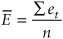Average error is the per-period average of cumulative error .

For example, the average error for the exponential smoothing forecast ( a = .30) is computed as follows (notice that the value of 11 was used for n because we used actual demand for the first-period forecast, resulting in no error, i.e., D 1 = F 1 = 37):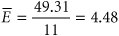The average error is interpreted similarly to the cumulative error. A positive value indicates low bias, and a negative value indicates high bias. A value close to zero implies a lack of bias.

Large +indicates that a forecast is biased low; large -indicates that a forecast is biased high .

Another measure of forecast accuracy related to error is mean squared error (MSE) . With MSE , each individual error value is squared, and then these values are summed and averaged. The last column in Table 15.8 shows the sum of the squared forecast errors (i.e., 376.04) for the PM Computer example forecast ( a = 0.30). The MSE is computed as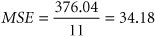As with other measures of forecast accuracy, the smaller the MSE , the better.

Table 15.9 summarizes the measures of forecast accuracy we have discussed in this section for the four example forecasts we developed in the previous section for PM Computer Services. The results are consistent for all four forecasts, indicating that for the PM Computer Services example data, a larger value of a is preferable for the exponential smoothing forecast. The adjusted forecast is more accurate than the exponential smoothing forecasts, and the linear trend is more accurate than all the others. Although these results are example specific, they do indicate how the different forecast measures for accuracy can be used to adjust a forecasting method or select the best method.

[Page 687]
##### Table 15.9. Comparison of forecasts for PM Computer Services

Forecast

MAD

MAPD (%)

EMSE

Exponential smoothing ( a = .30)

4.85

10.3

49.31

4.48

34.18

Exponential smoothing ( a = .50)

4.04

8.5

33.21

3.02

24.64

Adjusted exponential smoothing

3.81

8.1

21.14

1.92

21.58

( a = .50, b = .30)

Linear trend line

2.29

4.9

8.67

## Management Science Application: Demand Forecasting at National Car Rental

In 1993, due to large financial losses, National Car Rental was threatened with liquidation by its parent company, General Motors. The car rental industry had been in turmoil since the 1980s, when automobile manufacturers purchased almost all the major rental car companies and then flooded them with their excess auto production. The excess supply of cheap cars led to low prices, especially during periods of low demand, which eroded profits. When in the 1990s demand for cars increased, thus raising the cost of cars to the rental car companies, profits fell further.

Car rental companies rely largely on corporate clients that have large numbers of employees who travel. Rental car demand peaks at midweek, forcing car rental companies to turn away customers. They also have a large excess fleet that is idle on weekends, which, in turn , allows occasional renters, such as leisure customers, to book multiple reservations with no prepayment or cancellation or no-show penalty. This can result in no-shows that exceed 50% of reservations .

Prior to 1993, no demand forecasts had existed for National. To save itself from liquidation, National Car Rental committed over \$10 million to design and build a revenue management system that would manage capacity, pricing, and reservations. The system encompasses a number of management science and analytic models. A critical component of the system was the development of a comprehensive set of demand and revenue forecasts. Demand forecasts are made for the length of the rental period and the number of cars in use on a specific date. These demand forecasts are based on a combination of long- and short-term forecasts. The long- term forecast is a time series model with seasonal factors. The system generates forecasts for all days within a 60-day booking period. The system also generates forecasts for daily non-reservation customers and for no-shows.

The complete revenue management system produced immediate results, improving revenues by \$56 million in the first year alone. Rather than liquidate, General Motors sold National Car Rental in 1995 for \$1.2 billion.Source: M. K. Geraghty and E. Johnson, "Revenue Management Saves National Car Rental," Interfaces 27, no. 1 (JanuaryFebruary 1997): 10727.Introduction to Management Science (10th Edition)
ISBN: 0136064361
EAN: 2147483647
Year: 2006
Pages: 358

Similar book on Amazon

flylib.com © 2008-2017.
If you may any questions please contact us: flylib@qtcs.net